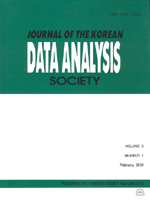상세검색
최근 검색어 전체 삭제
다국어입력
즐겨찾기0KCI등재 학술저널

# Confidence Intervals in Two Variance Components Mixed Model

• 등재여부 : KCI등재
• 2002.09
• 245 - 252 (8 pages)

In the two variance components mixed model, Y = Xβ + ZA + e , where Y(nx1) is a random vector, X(nxp) is a known matrix with rank p, β = ( β₁, β₂, ..., β_p ) , β₁, β₂, ..., β_p are unknown parameters. A and e are mutually independent normal random vectors with zero means, Z is known matrix. The confidence intervals for variance σ²_A by using Tukey-Williams method s is given by Ofversten (1993). This article shows that the probability ranges of confidence intervals for σ²_A are equals and whether greater than 1- α or not in the two variance components mixed model.

1. Introduction

2. Two Variance Components Mixed Model

3. Confidence Intervals

References